﻿ Global Energy Consumption Rates: Where is the Limit?

### Global Energy Consumption Rates: Where is the Limit?

Oleg P. DimitrievOPEN ACCESSPEER-REVIEWED

## Global Energy Consumption Rates: Where is the Limit?

Oleg P. DimitrievV.Lashkaryov Institute of Semiconductor Physics, Kiev, Ukraine

### Abstract

In this paper, energy consumption by the mankind is considered as a global process within the system of 'Sun-Earth-cosmic environment'. The upper limit for the energy consumption rate is estimated, provided that the thermodynamic equilibrium in this system is retained. This viewpoint leads to the following principles of energy consumption: (i) the energy should be consumed from the renewable sources only; (ii) the amount of the consumed energy should not exceed the amount of energy coming to the Earth. Three major sources of the renewable energy are considered: a direct incoming solar irradiation, a chemical energy due to products of photosynthesis and, finally, an outgoing infrared (IR) radiation from the Earth as a heated body. It is shown that only the first source gives the most effective way of energy consumption from the viewpoint of the global energy conversion. The ranges of materials suitable for construction of energy converters from the last source are briefly reviewed. It is discussed that the proper use of the outgoing IR radiation on the large scale can be helpful in controlling the climate changes. It is shown that the energy consumption rate which meets the global thermodynamic balance and expected technical facilities cannot exceed a limit of 1014W, which is approximately an order higher of the contemporary rate of energy consumption by the mankind. The conclusion is made that we should learn how to restrict the energy consumption rate in the near future.

### At a glance: Figures

123
Prev Next

• Dimitriev, Oleg P.. "Global Energy Consumption Rates: Where is the Limit?." Sustainable Energy 1.1 (2013): 1-6.
• Dimitriev, O. P. (2013). Global Energy Consumption Rates: Where is the Limit?. Sustainable Energy, 1(1), 1-6.
• Dimitriev, Oleg P.. "Global Energy Consumption Rates: Where is the Limit?." Sustainable Energy 1, no. 1 (2013): 1-6.

 Import into BibTeX Import into EndNote Import into RefMan Import into RefWorks

### 1. Introduction

Nowadays, search for energy sources for economy needs is one of the key issues of development of our civilization. On the other hand, the global strategy of energy consumption rates has not ever been developed or widely accepted yet. It is a common situation, when an average consumer is not even interested in such questions as ‘is there a limit of energy consumption rates?’ Or ‘can we take from the Nature more?’ It is evident that the world energy consumption increases more rapidly than the population growth during the last decades and this tendency is expected to be further increasing next years (Figure 1). Although the energy consumption rate is not mapped uniformly in different regions of the world, this general tendency persists. Even Eastern Europe, where the population decreases, demonstrates the continuing increase in the energy production and consumption (Figure 2). That means that the energy consumption per capita in general will continue to increase without visible tendency towards limitation in the near future. It should be noted that developing countries contribute now to the energy consumption rate more than industrial countries, with the average annual increase of energy consumption to be 3.5% versus 0.9%, respectively (Figure 3). However, since population in developing countries increases faster as well and because the absolute level of energy consumption in these countries is still low, the disruption in energy per capita by 5-6 times persists between the developing and industrial countries and it even continues to increase (Figure 4).

Figure 1. Comparison of the energy consumption and population growth in the world. The curves have been calculated based on the data of the International Energy Agency Report 2004

However, there are several signals indicating in favor that the energy consumption strategy should be assumed in the near future. One signal comes from the fact that the organic fuel supplies (oil and gas) are exhausted and will come to the end in a few tens of years, and even the coal reserves will run out faster than many believe [1, 2]. The second signal comes from the fact that the global climate is now sensitive to the increasing level of energy consumption and carbon dioxide production as a result of such kind of activity, respectively. As a result, more and more discussions appear in the literature concerning whether the decrease in overall energy demand should be achieved despite a growth in both population and volume of energy services [1, 3, 4, 5, 6], or the causal relationship between GDP and energy consumption will further persist [7, 8, 9].

Figure 2. Comparison of the energy consumption and population growth rates in different regions of the world, derived from the International Atomic Energy Agency data
Figure 3. Comparison of the energy consumption and population growth in (a) industrial and (b) developing countries. Calculated from data given in ref.
Figure 4. Energy per capita for the different groups of countries as a function of time. The difference in the growth tendency for the industrial and developing countries is reflected via the slope values (tg ф) of these lines. Calculated from data given in ref.

In this paper, the principles of the global energy consumption rates are considered from the viewpoint of thermodynamic balance between the Earth and the cosmic environment, which is necessary to maintain stable and unchanged conditions for proper functioning of the Earth biosphere. This viewpoint leads to the following principles: (i) The energy should be consumed from the renewable sources only; (ii) The amount of the consumed energy should not exceed the natural production of energy due to incoming solar radiation which gives rise to formation of organic fuel, etc. Sun is considered as a global supplier of the renewable energy on the Earth, leading to formation of all sources of the organic fuel through photosynthesis, as well as energy of wind, hydro-energy through local heating of the earth surface, melting of ice, etc. Nuclear energy is not included in this balance since nuclear materials are not renewable and, moreover, nuclear waste still represents a very dangerous factor for the biosphere.

Among various transformations of solar energy which can be consumed, there are three major energy fluxes to be considered, namely, a direct solar irradiation, an indirect solar energy through products of photosynthesis and, finally, a reemitted energy from the Earth after the heating of the planet and its re-emission as a black body radiation at the heated temperature. As to the first flux, it is considered that although the power of incoming solar irradiation at the earth surface is of the order of 1017W, only a small part of that, approximately 1014W, can be utilized by solar cells due to limitations in their efficiency, as well as limitation in the earth area suitable for their displacement. The second way of using the energy through products of photosynthesis can also yield a maximum consumption rate of 1014W which is equivalent to the energy power production by the biosphere. The total rate of human energy consumption is of the order of 1013W at the moment . With the average 1.5% annual increase of the energy consumption on the Earth it will take about 150 years to reach the maximal possible limit of 1014W. Finally, there is a large domain of the renewable energy resource in the form of the outgoing terrestrial radiation which could yield additional income and shift the above limit of consumption to higher values. The methods to develop this domain will be discussed below. However, such a shift in energy consumption can be accompanied with unpredictable changes in temperature regimes on the Earth surface. Therefore, the energy consumption limit should be postulated anyway as soon as possible in the near future.

### 2. Global Energy Balance on the Earth

Let us consider the Earth as a thermodynamic system, with Sun as the source of the external incoming energy and a cosmic environment as a sink for the dissipated outgoing energy. The solar radiation reaching the earth surface has an average power per square meter of about 230W/m2 and its spectrum extends from the UV to the IR, where the major part of energy falls in the visible range with the maximum at the wavelength of about 0.5μm (Figure 5). Eventually, the same amount of energy must be lost by the ‘Earth-atmosphere’ system if the internal energy of this system remains constant and the climate is unchanged. Therefore, the Earth emits the terrestrial radiation as a heated body. However, the spectral range of this outgoing radiation is shifted to the IR since the average temperature of the radiative body, i.e., the Earth, is 2880 K (Figure 5). Although the peak amount of photons of the outgoing terrestrial radiation (which takes place at the wavelength of ca. 10 μm) is much smaller as compared to the corresponding peak of the incoming solar radiation at 0.5μm, i.e., 7x10-3 versus 1.5x103W/(m2μm), respectively (calculated using the data from ref. ), the outgoing radiation has a much wider spectral range, so that the total amount of outgoing energy is equal to the incoming one in accordance with the thermodynamic balance of the planet.

On the other hand, the amount of photons incoming to and outgoing from the Earth is rather different according to the balance of the incoming and outgoing energy, i.e.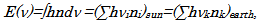where ni is the amount of photons with the frequency νi, h the Planck constant. It can be roughly evaluated this relationship as Nearth/Nsun=20, where Nearth is the average number of photons with the wavelength of 10μm emitted by the ‘Earth-atmosphere’ system and Nsun is the average number of photons with the wavelength of 0.5μm from the Sun.

Figure 5. Relationship between incoming solar (blue curve) and outgoing terrestrial (red curve) radiation. The magnitude of the terrestrial radiation is magnified by a factor of 500,000

However, there is a small disbalance between the incoming and outgoing energy, because a small part of photons from the Sun is stored in the form of chemical energy due to photosynthesis. This part of photons can be roughly evaluated taking into account the energy conversion efficiency of photosynthesis which is of the order of 0.1% (see section 3 for details) that results in only a single stored photon of the thousand ones that are coming from the Sun. As a result, the relationship of the incoming, stored and outgoing energies can be presented schematically in Figure 6. Since only one incoming photon of one thousand gives rise to formation of all organic minerals and fuel, such as coal, natural gas, and petroleum as the products of photosynthesis, it can be easy to understand that only a small part of the incoming solar energy is consumed by the mankind through the organic fuel reserves.

### 3.Renewable Sources of Energy

Figure 6 illustrates various energy sources available on the Earth. These sources consist of energy reserves, such as biomass and organic fuel, geothermal, solar and gravitation energy, nuclear reserves, whose total energy is indicated in Joules. Solar and gravitation energy produces energy fluxes, such as wind, tides, water streams, etc., whose total energy power is indicated in Watts, which can be used directly. As can be seen in Figure 6, the most energetic fluxes (~1017 W) are related to solar and terrestrial radiation, followed by wind , tides, and photosynthesis (~1014 W). Although the power of the last three fluxes is of the same order, only a small part of the wind’s and tide’s energy can be utilized due to their significant local instability and dissipation over the Earth. Therefore, we consider only three major energy fluxes, i.e., solar and terrestrial radiation and photosynthesis.

a. Photosynthesis

The energy flux utilized by photosynthesis, Porg, is of the order of 1014 W. This value can be calculated from the photosynthesis equation, i.e.(1)

The process described by Eq. (1) involves 4 electrons to be transferred; each of them requires the energy of 1.2eV per electron. Therefore, formation of an O2 molecule requires consumption of 4.8eV. However, quantum efficiency of the system is low and needs on average 10 (from 8 to 12) photons of the visible light (~2eV) to be adsorbed, which results in synthesis of one molecule of oxygen. Therefore, the total energy consumed by the photosynthetic system to produce one molecule of O2 will be approximately EO2 ~10 quanta x 2eV = 20eV. Green plants produce totally 1014kg of O2 annually; this corresponds to the oxygen mass rate production mO2=3x109g per second. The energy power necessary to produce O2, therefore, will be(2)

where M=32 is the molar mass of O2, NA the Avogadro constant. Using this result and the power of solar energy reaching the Earth surface which is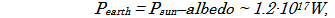where albedo is taken as 28%, one can evaluate the average efficiency of photosynthesis as(3)

The same value for ηorg can be also obtained via expression Porg = ηorg Searth∙230 W/m2, where an assumption is made that the plants cover the overall earth surface Searth. In fact, this assumption is rather crude, because there are large deserts, ice or mountain areas free of plants, but which can be overcompensated, however, by plant diversity in forests which occupy a few levels of space from the earth surface. The efficiency value (3) can also vary depending on the local conditions of growth of plant organisms, reaching, for example, up to 2% for water-plants grown in special pools .

It is natural to assume that the rate of energy consumption based on products of photosynthesis (gas, oil, coal, etc.) should not exceed the rate of energy formation stored in these organic products through photosynthesis, if the concept of energy consumption refers to these resources as the basic sources of energy. Thus, the energy consumption rate should be limited to Porg< 1014 W. This value is only one order of magnitude higher than the total power of all industrial energy producers currently on the Earth (about 1013 W, as reported in ref. ). The contemporary rate of energy consumption by the mankind can be calculated by using the data on total mass of burning of dry fuel equivalent to 51012 kg of carbon per year. The energy of carbon burning is 3.3107 J/kg which gives the energy power of 51012 W, which is consistent also with the annual energy consumption data of the order of 1017 W∙h reported in ref. .

Finally, the efficiency of conversion of the solar energy to some mechanical work or electricity by the indirect way through burning of the organic products of photosynthesis will be even smaller, assuming that power conversion efficiency of the burning process to the mechanical work or electricity is about 40%, and thus it yields only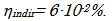(4)

Since power conversion efficiency of using a direct solar radiation is a few orders of magnitude higher, one can conclude that the products of photosynthesis, including organic fuel reserves (gas, oil and coal), are much expensive forms of the solar energy then the direct solar radiation itself.

The power of solar energy irradiating our planet is of the order of 1017W. That means that the theoretical maximum of the solar energy consumption can be of the same order, i.e., Psc~1017W, if the power conversion efficiency of the corresponding devices approaches 100%. There are limitations, however, because power conversion efficiency of the conventional solar cells is far lower, of the order of sc ~10%, and because these cells cannot be set over the whole surface of the Earth. For example, in order to produce energy comparable with that stored by photosynthesis, the area of the solar cells Ssc should be as much as 1% of the Earth surface Searth, which can be calculated from the following expressions with account of power conversion efficiency of the photosynthetic process (Eq.(3)):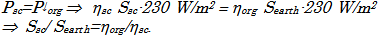(5)

One percent of the earth surface is rather large but reasonable value, which should not significantly affect the biosphere and cropland areas. For comparison, the total urban area on the Earth is approximately 3.4∙102km2 now ; it covers approximately 0.07 % of the Earth surface. To cover surface larger than the urban area by approximately ten times seems to be a challenging, but achievable task. In this case, the rate of the solar energy consumption by the mankind will be of the order of Psc~1014W. However, additional studies are needed to confirm that this value is acceptable and compatible with the life conditions on the Earth.

It is interesting to note that the accumulated amount of energy produced by solar cells from 1975 to 2010 has doubled every 2.5 years and now reached the level of almost 3·1010W . This exponential growth formally follows the Moor’s law  and if such a tendency will continue in the future, it is easy to calculate that the conventional upper level of energy production by solar cells of 1014W will be reached in the next 30 years.

Thus, the energy production rate through a direct consumption of the solar irradiation can reach practically a value one order of magnitude higher as compared with the contemporary production rate of energy through burning of organic fuel (~1013W, see previous section). In addition, power conversion efficiency of the direct conversion of solar energy by a modest solar cell (ηsc ~10%) is three orders of magnitude higher as compared with that of the indirect production of energy (see Eq.(4)). Thus, a direct consumption of the solar energy is more efficient than the apparent efficiency of burning of the carbon-containing products, such as oil, gas, coal and biomass.

There is a large part of IR energy, Pearth, which is reemitted from the earth surface in the form of the terrestrial radiation, because the Earth emits as the black body with the temperature of Tearth=2550 K. The amount of this energy is huge and comparable with that coming from the sun to the earth surface, due to the thermodynamic balance of the planet (see Figure 6),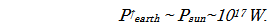There are several classes of materials that can be used as converters of this IR energy to electricity, such as pyroelectric and thermoelectric materials, gapless semiconductors, thermocouples, etc.; however, their proper application in respect to the terrestrial energy has not been developed yet. The advantages of the consumption of the terrestrial radiation are following. First, this radiation is highly scattered and therefore there is no need for surface orientation of the corresponding IR receivers or this orientation is not so critical as compared with the solar cells converting direct solar irradiation. Therefore, such IR receivers can occupy several levels upward from the earth surface without notable shadow effect. Second, these devices do not compete with green plants for the solar light, since they operate in the other spectral range. Below we consider three classes of materials which can be potentially used for the above energy domain.

It is well known that some semiconductors are gapless or have a narrow band gap smaller than 1eV (equivalent to ~0.8μm). Many of these semiconductor crystals have the band gap values that allow them to absorb in the near-IR range (Table 1). However, there are few candidates, i.e., HgSe, HgTe, HgxCd1-xTe, which can collect energy from the middle and even far IR range also. These last compounds, however, are toxic, and their application, therefore, are to be restricted. Nevertheless, development of these materials in the form of colloidal particles  packed in the inert matrix might overcome this problem. The advantage of the nanoparticle application is also due to opportunity to tune their range of absorption by simple changes of the particle size (Figure 7).

#### Table 1. Some semiconductor crystals absorbing IR radiationDownload asPowerPoint Slide

Veiw figureView current table in a new window

Figure 8. Examples of some organic compounds absorbing in the IR: a) donor-acceptor-donor D-π-A-π-D; b) TTF-σ-TCNQ; c) TTF-dithiolato metal complexes (R=(CH2)3, M=Ni; R=S(CH2)3S, M=Ni; R=Me, M=Ni; R=H, M=Pd) followed by their absorption spectra (Adapted from ref. )

The second class of materials which can be used for IR absorption consists of some organic compounds, such as metal-organic complexes, ionic dyes, extended π-conjugated chromophores, and donor–acceptor charge transfer chromophores which can absorb near-IR (NIR) light with the wavelengths up to 1m . Recent effort of chemists, however, resulted in development of a new series of donor-acceptor and donor-acceptor-donor D-π-A-π-D NIR compounds (Figure 8) whose absorption can be tuned within the wavelength region of 0.6-1.4μm . Even more exciting examples reperesent donor-acceptor compounds of tetrathiafulvalene-tetracyanoquinodimethane(TTF--TCNQ), fused porphyrin ribbons and TTF-dithiolato metal complexes, which demonstrate the absorption spectra extended to the middle-IR with the absorption maxima at 1.6, 2.9, and 4.6μm, respectively, where the thermo-excited electron transfer can be observed experimentally . Great opportunity can be expected also from the carbon materials and particularly graphene  which has a zero band gap and, therefore, can absorb photons from the whole IR range. Thus, design of energy converters based on some organic molecules which combine high stability and electronic absorption extended to the middle and far IR is an exciting and challenging problem.

The above types of the IR receivers produce an electron-hole pair upon absorption of an IR photon. Their advantage as compared with the solar cells operating in the visible spectrum is that the amount of IR photons is much larger as compared with photons of the visible light from the solar spectrum (see Figure 6); therefore, the IR devices can potentially collect more photons and produce a larger amount of electron-hole pairs as compared with the conventional solar cells operating in the visible region. On the other hand, the devices which can respond in the middle and far IR still should be developed to collect light quanta from a more extended spectral range.

The third class of materials perspective for harvesting IR radiation is thermoelectric materials. The thermoelectric energy conversion unit normally consists of two different (n- and p-type) semiconducting materials connected together in the form of a thermocouple; these materials are now actively developed , although some skepticism concerning their perspective in the energy solution domain exists . It should be noted that the advantage of thermoelectric devices is that these can consume IR energy of practically all wavelengths and they do not have a quantum threshold restriction from which the device becomes active. However, a sufficiently large thermal gradient is needed for their effective work.

There is a question which part of the IR spectrum is most suitable to use. If, for example, the corresponding devices will collect IR energy which normally leaves the Earth through the transparency window of the atmosphere (i.e., within the wavelength range of 8-13μm or energy range 0.1-0.15eV, Figure 7) their work can provoke the increase in the temperature regime at the earth surface. On the other hand, if the IR converters will collect energy in the region of absorption of greenhouse gases and scatter it in the transparency window, their application could weaken the greenhouse effect and decrease the temperature, respectively. Therefore, the use of the above converters could help in controlling the climate changes. However, such effects, if any, can be expected only if the converters of IR energy will be applied on the large scale.

There is no estimate at the moment how much terrestrial radiation can be consumed, since the respective energy converters are at the beginning of their development. It should be noted that power conversion efficiency of the respective devices which convert IR radiation to electricity is very low, especially without cooling. This makes their work under environmental conditions inefficient, since the environment temperatures are very close to the temperature of the emitting source. It is known, for example, that power conversion efficiency of thermoelectric devices is of the order of few percents . The same order of magnitude is typical for the best photovoltaic cells based on organic materials. Therefore, if to take an optimistic efficiency of ηsc ~1% for a conventional IR device constructed of organic materials, it would be hard to reach the above limit of 1014 W of the energy collection rate, and this could be achieved only by a multilevel arrangement of the collecting cells. Thus, the value of 1014 W should be addressed as the upper limit of the energy consumption rate upon use of all renewable sources of energy.

### 4.Concluding Remarks

Based on the above analysis of consumption rates from different sources of renewable energy, one can conclude that the energy consumption rate limit should be set as a necessary demand in the near future to conserve thermodynamic balance on the Earth. Such a limit, if based on the production of energy through burning of organic fuel, conversion of a direct solar and outgoing terrestrial radiation as the major energy contributors on the Earth, was determined as 1014W and it exceeds the contemporary rate of energy consumption by only one order of magnitude. It is easy to calculate when this limit will be reached from now based on the different scenarios of energy consumption, according to the formulae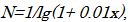(6)

where x is the annual increase in the energy consumption rate in percents, N the time interval in years.

Assuming as an example the annual increase of the energy consumption rate to be 4% which is typical of the developing countries, the above limit will be reached in the next 60 years. Fortunately, in IAEA-2009 reference scenario world primary energy demand is projected to increase by 1.5% per year between 2007 and 2030 . This will result in that the indicated energy consumption rate limit will be reached in approximately 155 years. On the other hand, the exponential growth of energy production by solar cells will result in reaching the above limit in the next 30 years, if such a production will continue to follow the Moore’s law in the future . Anyway, our and next generations should be alerted and learn how to restrict the energy consumption rate in the near future. Necessary political and economical steps should be undertaken towards the necessity of the energy consumption constraints to keep the living conditions on the Earth in accordance with the global natural thermodynamic balance.References

### List of abbreviations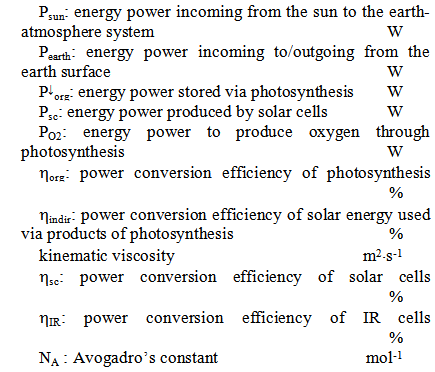### References

  Shafiee S., Topal E., “When will fossil fuel reserves be diminished?” Energy Policy, 37, 181-189, 2009.In article CrossRef  Heinberg R., Fridley D., “The end of cheap coal”, Nature, 468, 367-369, 2010.In article CrossRef PubMed  Deng Y.Y., Blok K, van der Leun K., “Transition to a fully sustainable global energy system”, Energy Strategy Reviews, 1, 109-121, 2012.In article CrossRef  Steinberger J. K., Roberts J. T., “From constraint to sufficiency: The decoupling of energy and carbon from human needs, 1975-2005”, Ecological Economics, 70, 425-433, 2010.In article CrossRef  Mehrara M., “Energy consumption and economic growth: The case of oil exporting countries”, Energy Policy, 35, 2939-2945, 2007.In article CrossRef  Lise W., Van Montfort K., “Energy consumption and GDP in Turkey: Is there a co‐integration relationship?” Energy Economics, 29, 1166-1178, 2007.In article CrossRef  Lee C.C., “The causality relationship between energy consumption and GDP in G-11 countries revisited”, Energy Policy, 34, 1086-1093, 2006.In article CrossRef  Soytas U, Sari R., “Energy consumption and GDP: causality relationship in G-7 countries and emerging markets”, Energy Economics, 25, 33-37, 2003.In article CrossRef  Crompton P., Wu Y., “Energy consumption in China: past trends and future directions”, Energy Economics, 27, 195-208, 2005.In article CrossRef  Aggarwal S.K., Gupta A.K., Lilley D.G., “Terrestrial energy”, Aerospace America, 12, 70, 2006.In article  Energy, Electricity and Power Energy Estimates in the Period up to 2030, Reference data series No.1, IAEA, Vienna, 2009.In article  Abbot C.G., “Terrestrial temperature and atmospheric absorption”, Proc. Natl. Acad. Sci. USA, 4, 104-106, 1918.In article CrossRef PubMed  Archer C.L., Jacobson M.Z., “Evaluation of global wind power”, J. Geophys. Res., 110, D12110, 2005.In article CrossRef  Alexeev V.V., Chekarev K.V., Solar energetics, Nauka, Moscow, 48-50, 1991.In article  Kondratyev K.Ya., “Key aspects of global climate change”, Energy and Environment, 15, 467-501, 2004.In article CrossRef  Demographia world urban areas & population projections. Edition 6.1, 1-128, 2010 (www.demographia.com/db-worldua.pdf).In article  World Solar Photovoltaics Production, 1975-2010, Earth Policy Institute, 1-3, 2011.In article  Norris D.J., Aydil E.S., “Getting Moore from solar cells”, Science, 338, 625-626, 2012.In article CrossRef PubMed  Harrison M.T., Kershaw S.V., Burt M.G., Rogach A.L., Kornowski A., Eychmüller A., Weller H., “Colloidal nanocrystals for telecommunications. Complete coverage of the low-loss fiber windows by mercury telluride quantum dots”, Pure Appl. Chem., 72, 295-307, 2000.In article CrossRef  Qian G., Wang Z.Y., “Near-infrared organic compounds and emerging applications”, Chem. Asian J., 5, 1006-1029, 2010.In article CrossRef PubMed  Perepichka D. F., Bryce M. R., “Molecules with exceptionally small HOMO–LUMO gaps”, Angew. Chem. Int. Ed., 44, 5370-5373, 2005.In article CrossRef PubMed  Dawlaty J.M., Shivaraman S., Strait J., George P., Chandrashekhar M., Rana F., Spencer M.G., Veksler D., Chen Y., “Measurement of the optical absorption spectra of epitaxial graphene”, Appl. Phys. Lett., 93, 13195, 2008.In article CrossRef  Li J.F., Liu W.S., Zhao L.D., Zhou M., “High-performance nanostructured thermoelectric materials”, NPG Asia Mater., 2, 152-158, 2010.In article CrossRef  Vining C.B., “An inconvenient truth about thermoelectric”, Nature Mater., 8, 83-85, 2009.In article CrossRef PubMed